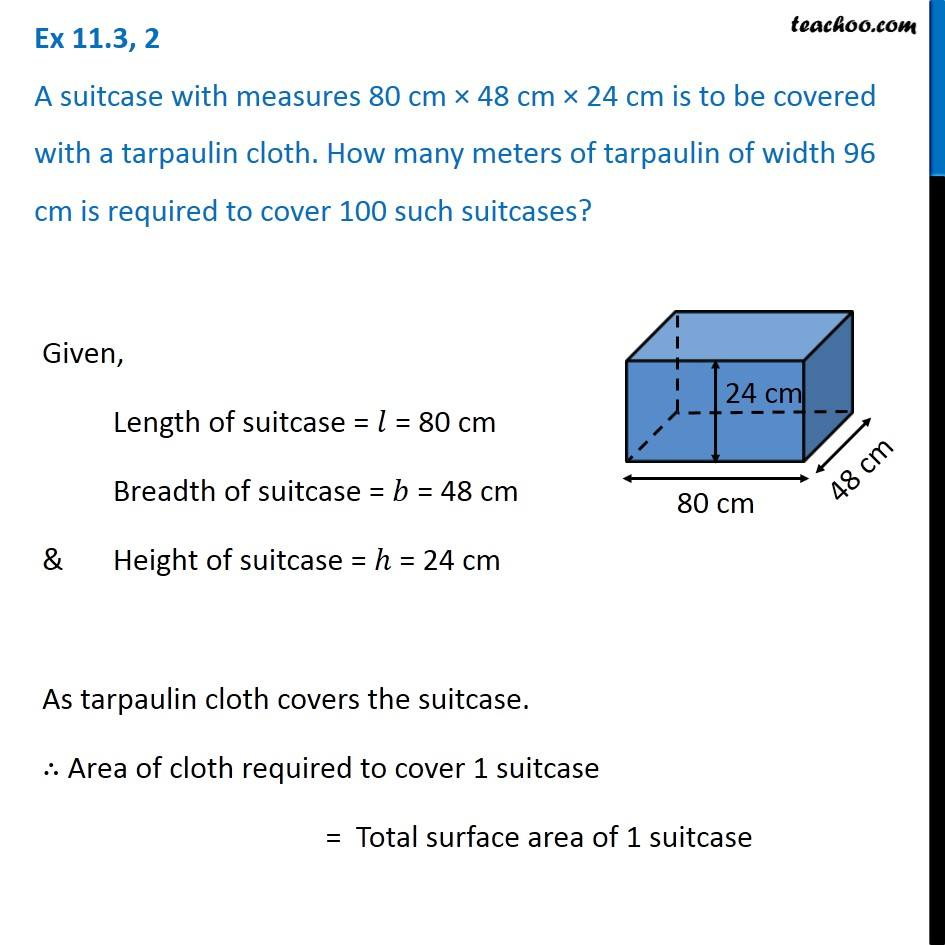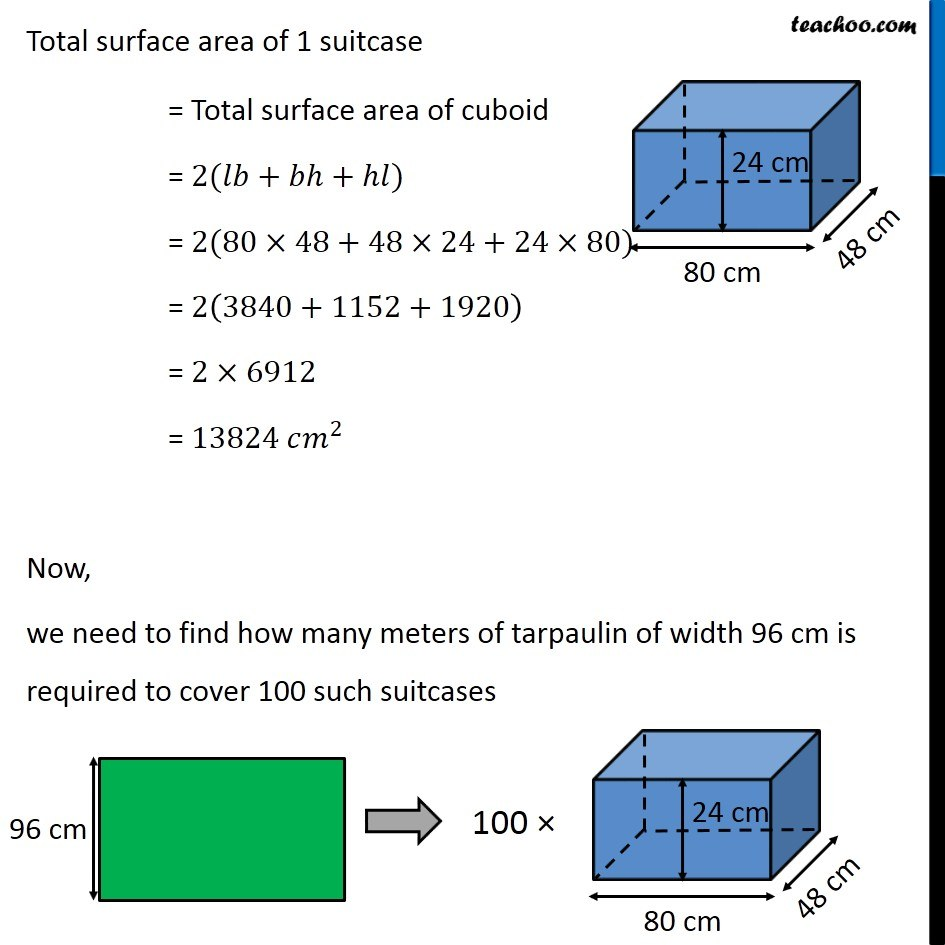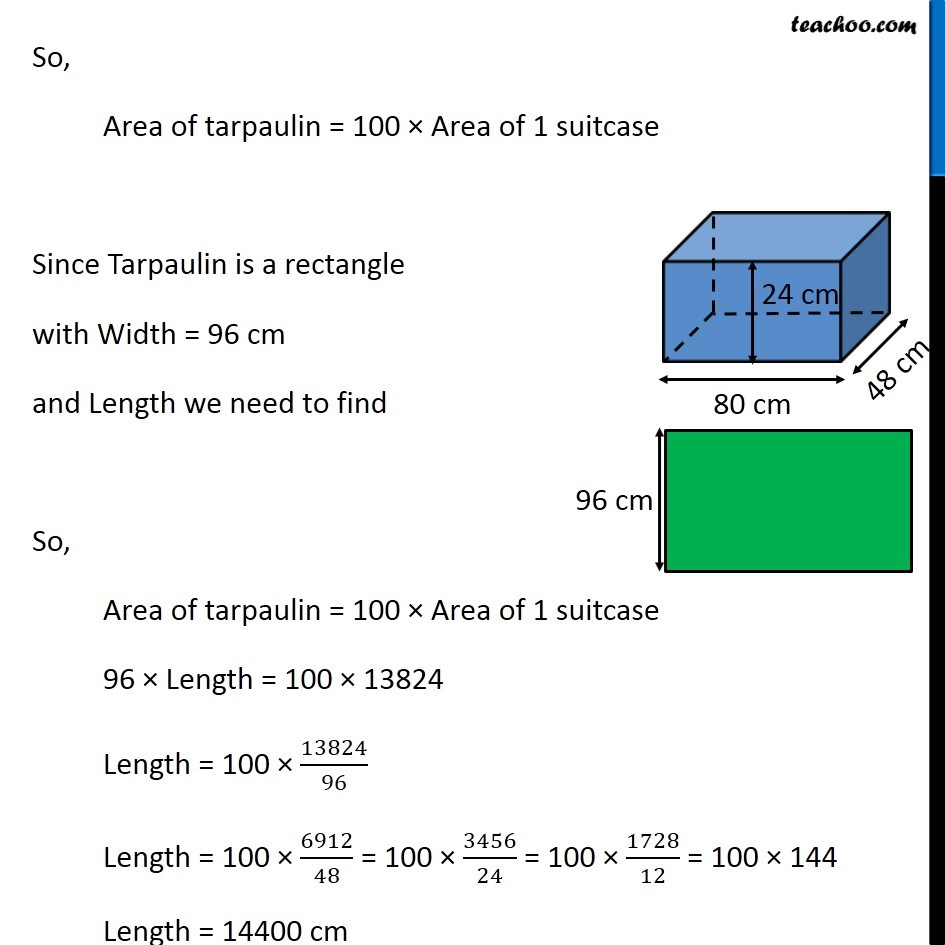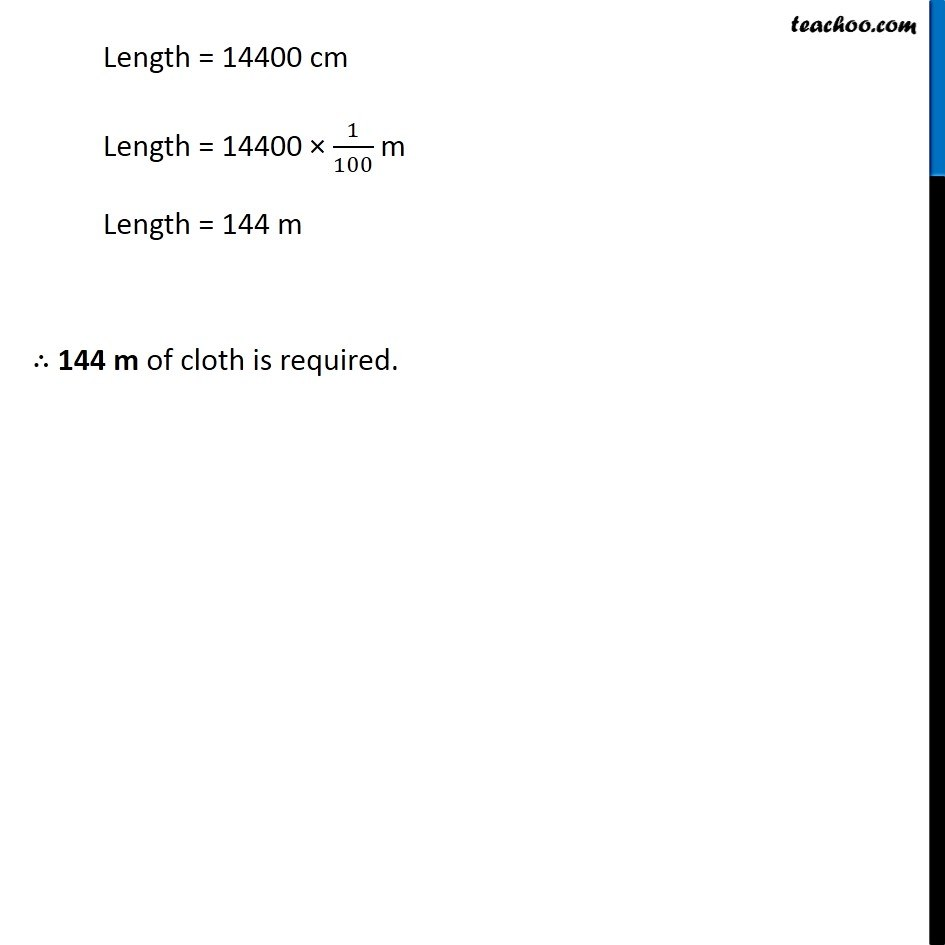Surface Area of Cuboid

Chapter 11 Class 8 Mensuration
Concept wiseIntroducing your new favourite teacher - Teachoo Black, at only ₹83 per month

### Transcript

Ex 11.3, 2 A suitcase with measures 80 cm × 48 cm × 24 cm is to be covered with a tarpaulin cloth. How many meters of tarpaulin of width 96 cm is required to cover 100 such suitcases? Given, Length of suitcase = 𝑙 = 80 cm Breadth of suitcase = 𝑏 = 48 cm & Height of suitcase = ℎ = 24 cm As tarpaulin cloth covers the suitcase. ∴ Area of cloth required to cover 1 suitcase = Total surface area of 1 suitcase Total surface area of 1 suitcase = Total surface area of cuboid = 2(𝑙𝑏+𝑏ℎ+ℎ𝑙) = 2(80×48+48×24+24×80) = 2(3840+1152+1920) = 2×6912 = 13824 𝑐𝑚^2 Now, we need to find how many meters of tarpaulin of width 96 cm is required to cover 100 such suitcases So, Area of tarpaulin = 100 × Area of 1 suitcase Since Tarpaulin is a rectangle with Width = 96 cm and Length we need to find So, Area of tarpaulin = 100 × Area of 1 suitcase 96 × Length = 100 × 13824 Length = 100 × 13824/96 Length = 100 × 6912/48 = 100 × 3456/24 = 100 × 1728/12 = 100 × 144 Length = 14400 cm Length = 14400 cm Length = 14400 × 1/100 m Length = 144 m ∴ 144 m of cloth is required.